Test: Shapes - 2

# Test: Shapes - 2

Test Description

## 10 Questions MCQ Test Mathematics for Class 1 | Test: Shapes - 2

Test: Shapes - 2 for Class 1 2023 is part of Mathematics for Class 1 preparation. The Test: Shapes - 2 questions and answers have been prepared according to the Class 1 exam syllabus.The Test: Shapes - 2 MCQs are made for Class 1 2023 Exam. Find important definitions, questions, notes, meanings, examples, exercises, MCQs and online tests for Test: Shapes - 2 below.
Solutions of Test: Shapes - 2 questions in English are available as part of our Mathematics for Class 1 for Class 1 & Test: Shapes - 2 solutions in Hindi for Mathematics for Class 1 course. Download more important topics, notes, lectures and mock test series for Class 1 Exam by signing up for free. Attempt Test: Shapes - 2 | 10 questions in 15 minutes | Mock test for Class 1 preparation | Free important questions MCQ to study Mathematics for Class 1 for Class 1 Exam | Download free PDF with solutions
 1 Crore+ students have signed up on EduRev. Have you?
Test: Shapes - 2 - Question 1

### Father bought a pizza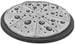. In which shape is it?

Detailed Solution for Test: Shapes - 2 - Question 1

A pizza is in the shape of a circle.

Test: Shapes - 2 - Question 2

### What is the shape of the shaded part in the figure?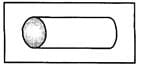Detailed Solution for Test: Shapes - 2 - Question 2

The shaded part is in the shape of a circle.

Test: Shapes - 2 - Question 3

### How many triangles are there in the figure given?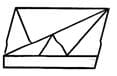Detailed Solution for Test: Shapes - 2 - Question 3

The triangles in the given figure are 1,  2, 3, 4, 5, 6 = 6 triangles.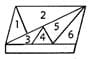3 + 4 = 1 triangle 1 + 2 = 1 triangle 3 + 4 + 5 = 1 triangle + 4 + 5 + 6 = 1 triangle
∴ Number of triangles = 6 + 1 + 1 + 1 + 1 = 10 triangles.

Test: Shapes - 2 - Question 4

Which of the following looks like a cylinder?

Detailed Solution for Test: Shapes - 2 - Question 4

A cool drink can is in the shape of a cylinder.

Test: Shapes - 2 - Question 5

Mother blew some balloons on your birthday. In which shape were they?

Detailed Solution for Test: Shapes - 2 - Question 5

Balloons are in the shape of an oval.

Test: Shapes - 2 - Question 6

Which of the following is matched correctly?

Detailed Solution for Test: Shapes - 2 - Question 6

A ball is in the shape of a sphere.

Test: Shapes - 2 - Question 7

Rectangle has ___________ sides and ____________ corners.

Detailed Solution for Test: Shapes - 2 - Question 7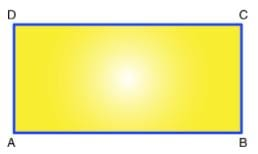A rectangle is a 2D shape in geometry, having 4 sides and 4 corners. Its two sides meet at right angles. Thus, a rectangle has 4 angles, each measuring 90 ̊.

Test: Shapes - 2 - Question 8

Rohan has some chocolate bars. In which plate will he put them?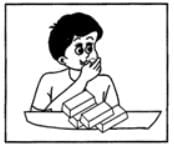Detailed Solution for Test: Shapes - 2 - Question 8

Chocolate bars are in the shape of a cuboid

Test: Shapes - 2 - Question 9

Suraj has some laddus. In which shape are they?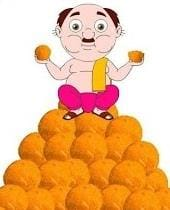Detailed Solution for Test: Shapes - 2 - Question 9

Laddus are in the shape of a sphere.

Test: Shapes - 2 - Question 10

Which of the following is a square?

Detailed Solution for Test: Shapes - 2 - Question 10

The figure in option (a) is a square.

## Mathematics for Class 1

10 videos|36 docs|23 tests
 Use Code STAYHOME200 and get INR 200 additional OFF Use Coupon Code
Information about Test: Shapes - 2 Page
In this test you can find the Exam questions for Test: Shapes - 2 solved & explained in the simplest way possible. Besides giving Questions and answers for Test: Shapes - 2, EduRev gives you an ample number of Online tests for practice

## Mathematics for Class 1

10 videos|36 docs|23 tests